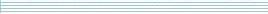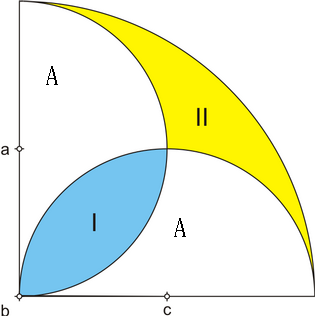| home      | people     | research     | publications    | seminars    | events     | contactCommunication Networks   | Systems Biology   | Hybrid Systems    | Machine Learning   | Dynamics & Interaction### Schools Mathematics Grand Challenge

Week three's Puzzles

Problem 7:

The solution was:

We'll use B for the amount of cola in a bottle and G as the amount in a glass. When John fills 6 glasses using 6 bottles, he has one bottle left over,

6B = 6G + 1B,

so 5B = 6G, or B = 6/5 G. Now, we want to know what 36 bottles will fill. 36B = 36*6/5 G = 43.2 G. So we can fill 43 whole bottles and have a little left at the end.

Problem 8:

The solution was:

The area of a circle is pi*r*r, where pi is 3.14159... and r is the radius of the circle. The area of a semicircle is just half this, or pi*r*r/2, and the area of a quarter circle is one quarter this, pi*r*r/4.Let the r be the distance from a to b. Then the area of the semicircle centered at a is pi*r*r/2, and the area of the semicircle centered at c is the same. The area of the big quarter circle is pi*2r*2r/4 = pi*r*r, because its radius is twice as big as the small circles.

We've marked in the area of each white bit as A. The area of the semicircles is:

pi*r*r/2 = I + A

So, the area of I = pi*r*r/2 - A. The area of the big quarter circle is:

pi*r*r = I + II + A + A

if we subtract off the area of a semicircle circle, then we get,

pi*r*r - pi*r*r/2 = I + II + A + A - I - A

or

pi*r*r/2 = II + A

so II = pi*r*r/2 - A. This means that the area of I and II are the same, so the ratio of the areas is 1.

Problem 9:

The solution was:

When the driver drives from the start to the end, suppose he drives a distance U uphill, D downhill and F on the flat. It takes him 2 hours, so:

U/112 + F/126 + D/144 = 2

on the way back again, he is going the opposite direction, so the uphill bits become downhill and the downhill bits become uphill. It takes him 2 hours 20 minutes, which is two and a third hours:

U/144 + F/126 + D/112 = 7/3

We want to know how long the track is, which is U+F+D. This looks a bit like simultaneous equations, but we have three things we don't know, and only two equations, so we can't actually find U, F and D, but we can find their sum. If we add the two equations, we get:

U/112 + U/144 + F/126 + F/126 + D/144 + D/112 = 2 + 7/3

Then we add up all the fractions: 1/112 + 1/144 = 1/63, 1/126 + 1/126 = 1/63 and 2+7/3 = 13/3. So we get:

(U + F + D)/63 = 13/3,

or U + F + D = 63*13/3 = 273, so the answer is 273 kilometres.